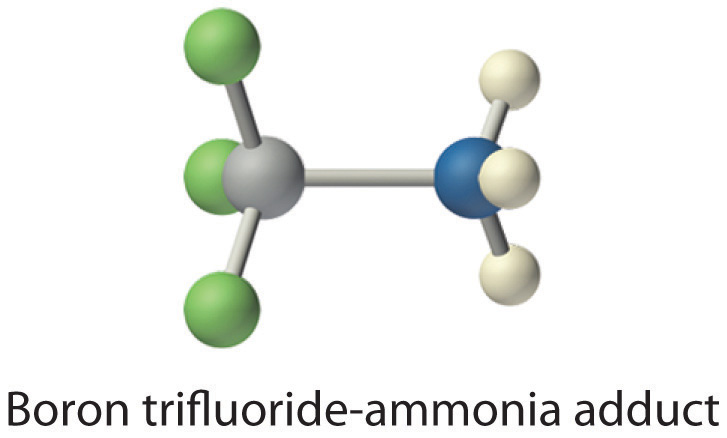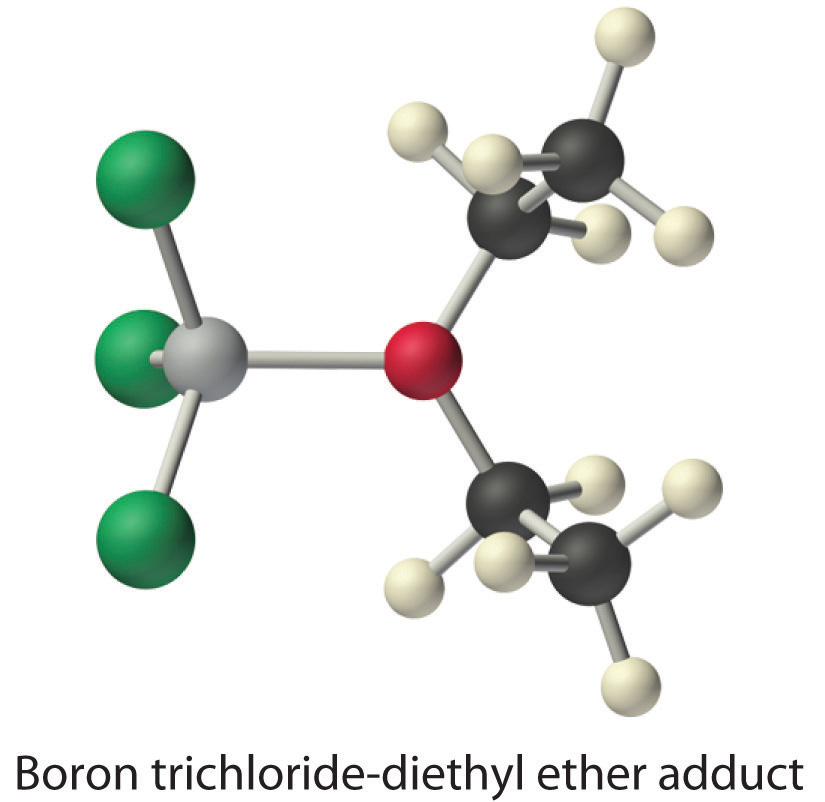This is “Lewis Acids and Bases”, section 8.7 from the book Principles of General Chemistry (v. 1.0M). For details on it (including licensing), click here.

For more information on the source of this book, or why it is available for free, please see the project's home page. You can browse or download additional books there. To download a .zip file containing this book to use offline, simply click here.

Has this book helped you? Consider passing it on:
Creative Commons supports free culture from music to education. Their licenses helped make this book available to you.
DonorsChoose.org helps people like you help teachers fund their classroom projects, from art supplies to books to calculators.

## 8.7 Lewis Acids and Bases

### Learning Objective

1. To identify Lewis acids and bases.

As you learned in Chapter 4 "Reactions in Aqueous Solution", the Brønsted–Lowry concept of acids and bases defines a base as any species that can accept a proton, and an acid as any substance that can donate a proton. Lewis proposed an alternative definition that focuses on pairs of electrons instead.

A Lewis baseAny species that can donate a pair of electrons. is defined as any species that can donate a pair of electrons, and a Lewis acidAny species that can accept a pair of electrons. is any species that can accept a pair of electrons. All Brønsted–Lowry bases (proton acceptors), such as OH, H2O, and NH3, are also electron-pair donors. Thus the Lewis definition of acids and bases does not contradict the Brønsted–Lowry definition. Rather, it expands the definition of acids to include substances other than the H+ ion.

Electron-deficient moleculesA compound that has less than an octet of electrons around one atom., such as BCl3, contain less than an octet of electrons around one atom and have a strong tendency to gain an additional pair of electrons by reacting with substances that possess a lone pair of electrons. Lewis’s definition, which is less restrictive than either the Brønsted–Lowry or the Arrhenius definition, grew out of his observation of this tendency.

### Note the Pattern

Electron-deficient molecules (those with less than an octet of electrons) are Lewis acids.

A general Brønsted–Lowry acid–base reaction can be depicted in Lewis electron symbols as follows:The proton (H+), which has no valence electrons, is a Lewis acid because it accepts a lone pair of electrons on the base to form a bond. The proton, however, is just one of many electron-deficient species that are known to react with bases. For example, neutral compounds of boron, aluminum, and the other group 13 elements, which possess only six valence electrons, have a very strong tendency to gain an additional electron pair. Such compounds are therefore potent Lewis acids that react with an electron-pair donor such as ammonia to form an acid–base adductThe product of a reaction between a Lewis acid and a Lewis base with a coordinate covalent bond., a new covalent bond, as shown here for boron trifluoride (BF3):The bond formed between a Lewis acid and a Lewis base is a coordinate covalent bond because both electrons are provided by only one of the atoms (N, in the case of F3B:NH3). After it is formed, however, a coordinate covalent bond behaves like any other covalent single bond.

Species that are very weak Brønsted–Lowry bases can be relatively strong Lewis bases. For example, many of the group 13 trihalides are highly soluble in ethers (R–O–R′) because the oxygen atom in the ether contains two lone pairs of electrons, just as in H2O. Hence the predominant species in solutions of electron-deficient trihalides in ether solvents is a Lewis acid–base adduct. A reaction of this type is shown in Equation 8.19 for boron trichloride and diethyl ether:Many molecules with multiple bonds can act as Lewis acids. In these cases, the Lewis base typically donates a pair of electrons to form a bond to the central atom of the molecule, while a pair of electrons displaced from the multiple bond becomes a lone pair on a terminal atom. A typical example is the reaction of the hydroxide ion with carbon dioxide to give the bicarbonate ion, as shown in Equation 8.21. The highly electronegative oxygen atoms pull electron density away from carbon, so the carbon atom acts as a Lewis acid. Arrows indicate the direction of electron flow.### Example 9

Identify the acid and the base in each Lewis acid–base reaction.

1. BH3 + (CH3)2S → H3B:S(CH3)2
2. CaO + CO2 → CaCO3
3. BeCl2 + 2 Cl → BeCl42−

Given: reactants and products

Asked for: identity of Lewis acid and Lewis base

Strategy:

In each equation, identify the reactant that is electron deficient and the reactant that is an electron-pair donor. The electron-deficient compound is the Lewis acid, whereas the other is the Lewis base.

Solution:

1. In BH3, boron has only six valence electrons. It is therefore electron deficient and can accept a lone pair. Like oxygen, the sulfur atom in (CH3)2S has two lone pairs. Thus (CH3)2S donates an electron pair on sulfur to the boron atom of BH3. The Lewis base is (CH3)2S, and the Lewis acid is BH3.
2. As in the reaction shown in Equation 8.21, CO2 accepts a pair of electrons from the O2− ion in CaO to form the carbonate ion. The oxygen in CaO is an electron-pair donor, so CaO is the Lewis base. Carbon accepts a pair of electrons, so CO2 is the Lewis acid.
3. The chloride ion contains four lone pairs. In this reaction, each chloride ion donates one lone pair to BeCl2, which has only four electrons around Be. Thus the chloride ions are Lewis bases, and BeCl2 is the Lewis acid.

Exercise

Identify the acid and the base in each Lewis acid–base reaction.

1. (CH3)2O + BF3 → (CH3)2O:BF3
2. H2O + SO3 → H2SO4

Answer:

1. Lewis base: (CH3)2O; Lewis acid: BF3
2. Lewis base: H2O; Lewis acid: SO3

### Summary

A Lewis acid is a compound with a strong tendency to accept an additional pair of electrons from a Lewis base, which can donate a pair of electrons. Such an acid–base reaction forms an adduct, which is a compound with a coordinate covalent bond in which both electrons are provided by only one of the atoms. Electron-deficient molecules, which have less than an octet of electrons around one atom, are relatively common. They tend to acquire an octet electron configuration by reacting with an atom having a lone pair of electrons.

### Key Takeaway

• The acid-base behavior of many compounds can be explained by their Lewis electron structures.

### Conceptual Problems

1. Construct a table comparing how OH, NH3, H2O, and BCl3 are classified according to the Arrhenius, the Brønsted–Lowry, and the Lewis definitions of acids and bases.

2. Describe how the proton (H+) can simultaneously behave as an Arrhenius acid, a Brønsted–Lowry acid, and a Lewis acid.

3. Would you expect aluminum to form compounds with covalent bonds or coordinate covalent bonds? Explain your answer.

4. Classify each compound as a Lewis acid or a Lewis base and justify your choice.

1. AlCl3
2. (CH3)3N
3. IO3
5. Explain how a carboxylate ion (RCO2) can act as both a Brønsted–Lowry base and a Lewis base.

### Numerical Problems

1. In each reaction, identify the Lewis acid and the Lewis base and complete the reaction by writing the products(s).

1. (CH3)2O + AlCl3
2. SnCl4 + 2 Cl
2. Use Lewis dot symbols to depict the reaction of BCl3 with dimethyl ether [(CH3)2O]. How is this reaction similar to that in which a proton is added to ammonia?

### Answer

1. AlCl3 is the Lewis acid, and the ether is the Lewis base; AlCl3 + (CH3)2O → AlCl3·O(CH3)2
2. SnCl4 is the Lewis acid, and chloride is the Lewis base; SnCl4 + 2 Cl → SnCl62−